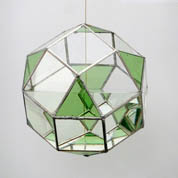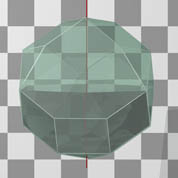Mathematics on the "Ritratto di Frà Luca Pacioli"

In the Museo e Gallerie di Capodimonte in Napoli (Italy) one can admire this "Ritratto di Frà Luca Pacioli".
The only thing that is certain is that the central person is Frà Luca Pacioli, one of the most famous mathematicians from the Renaissance period. The painter is unknown, although some people are convinced the painter is Jacopo de' Barbari. Other people think that the painting isn't the work of one artist. As to the other person on the painting, some people suppose it's Guidobaldo, Duke of Urbino, other people are convinced it's the famous painter Albrecht Dürer.

A very interesting paper about the historical and mathematical elements was published by Nick Mackinnon: "The portrait of Fra Luca Pacioli", The Mathematical Gazette, 77 (1993) pp. 130 - 219.

The closed book on the table is supposed to be the "Summa de arithmetica geometria proportioni et proportionalita", written by Pacioli. A regular dodecahedron is placed onto this book. The open book is book XIII from Euclid's Elements. Pacioli clearly is exposing a theorem to one of his pupils. Book XIII treats the Platonic solids or regular polyhedra.

The drawing on the slate occurs in proposition XIII.12, although Mackinnon mentions this drawing is also linked to other propositions concerning the regular dodecahedron and icosahedron.

The rhombicuboctahedron on the "Ritratto di Frà Luca Pacioli"

The object suspended in the top left is a "rhombicuboctahedron" (RCO), one of the thirteen semi-regular solids, also called the Archimedean solids. All the faces are regular polygons, but not all of the same kind. The suspended object seems to be made of glass plates and it is half filled with water. The painter has captured the reflections and refractions in an extraordinary way and Mackinnon attributes this part of the ritratto to Leonardo da Vinci himself!A rhombicuboctahedron (RCO) is a semi-regular polyhedron with 26 faces (18 squares and 8 equilateral triangles), 24 vertices and 48 edges. Each vertex belongs to three squares and one equilateral triangle. On these images a RCO is represented in the usual orientation.Click to enlarge However, the RCO on the painting has a different orientation. This orientation easily can be derived by viewing the object from the right side. The diagonals of the square in the centre are horizontally en vertically oriented as can be seen from the level of the water. This is a valuable help to determine the point on the upper triangle where the suspension string has been attached.We rotate the RCO as seen on the ritratto over -90 degrees around the vertical axis and we suppose the length of the edges of the RCO equals 1.The length of the red segments equals 1, that of the blue segments equals sqrt(2)/2 and the length of the green segments equals sqrt(3)/2. This is the real length of the altitude in the triangles. The length of CB is 1/2. The sine of the angle alpha equals sqrt(2/3). Using the sine rule in triangle SDB we obtain SB=(2*sqrt(3)-sqrt(6))/2 and at last SA=(sqrt(6)-sqrt(3))/2. The suspension point is situated on the altitude approximately 0.3587 from A.As to the material construction of a RCO as seen on the Ritratto it's worth to notice that, if the length of the edges of the RCO is 10 cm, then the volume is (12 + 10*sqrt(2))/3 litres or approximately 8.7 litres. The water that is needed to fill half of this RCO has a weigth of more than 4 kg!
 In May 2011 Claude Boehringer, an artist working with different materials, produced a first glass model of the RCO, filled it half with water and suspended it in the same orientation as the model on the ritratto.Herman Serras, March 2001 (updated May 2011)